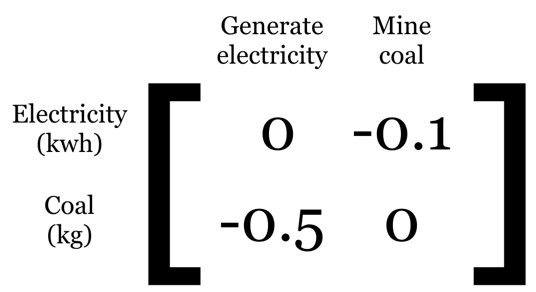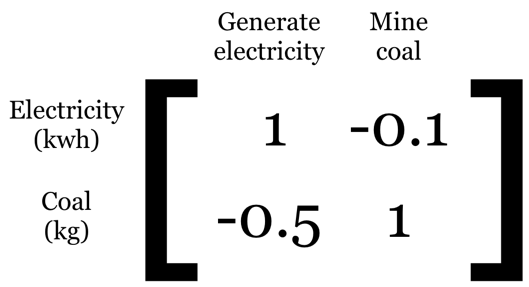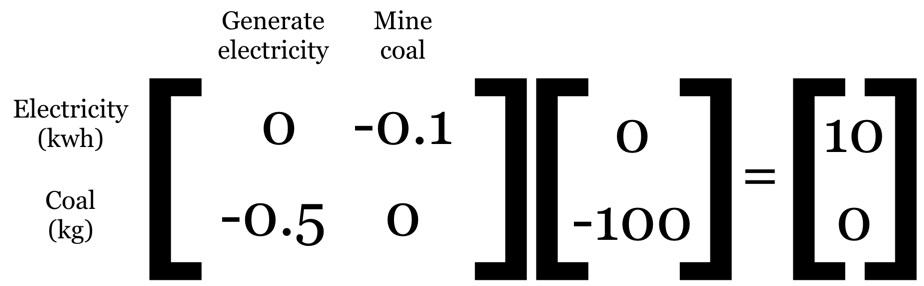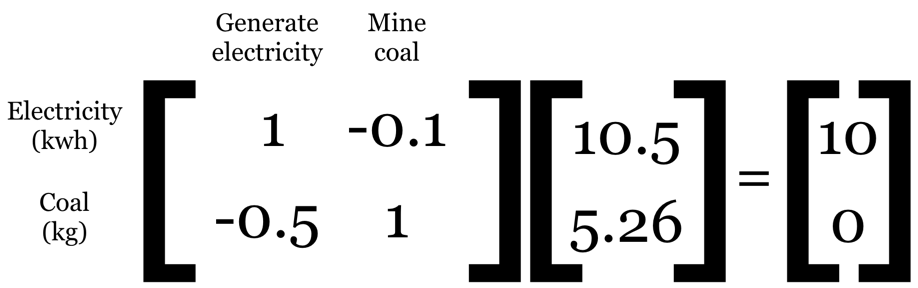# Diagonals are a social construct

• Tue 09 October 2018
• mathI recently made a fool of myself by claiming that a matrix with zeros on one of its diagonals is not invertible, which is very, very wrong. I was participating in a discussion on what value should be on the diagonal of what I would call the technosphere matrix - the matrix which gives our linear model of the industrial world. Normally, I would link to this discussion - I am not shy about admitting mistakes or looking silly, you can find a picture of me dressed as a colorful princess on this blog, and as the middle-aged father of four I make many mistakes every day - but unfortunately the discussion list is private.

## Matrix formulations

There are two different ways to write the fundamental matrix equation for life cycle assessment (LCA). The IO form:

\begin{equation*} (I - A)^{-1} diag( f ) \end{equation*}

and the general form:

\begin{equation*} A^{-1} diag( f ) \end{equation*}

There are also some other matrices in the full LCA calculation, but they don't matter here. These two formulations have both been used for a while, though (I - A) was used first, by Leontieff (Леонтьев, it's spelled multiple ways in English) in the development of Input-Output methodology.

## What number should be on the diagonal?

In both forms, the technosphere matrix ((I - A) or just A) provides a complete description of how we model our complex, interdependent, ever-changing global economy. The columns of the technosphere matrix represent activities, which have inputs and outputs. A common practice is for each activity to produce a single reference product, whose production is the reason that we are modelling this activity. However, this is not a hard limit - some activities can produce multiple products, and some can produce none. The rows of the technosphere matrix are products. Activities are unitless, but products have units of mass, energy, area, etc.

A second common practice is to align the rows and columns so that the activities and reference products are in the same order, and so the amount of each reference product produced by each activity appears on the diagonal. A final community standard is to normalize each activity to produce one of its reference product, whatever that may be.

If we follow all these conventions, what number should be on the diagonal? As long as the technosphere matrix is not singular, the linear algebra library will give us an answer. However, we want a bit more than just an answer - we need something that fits into our mental model of how our system is constructed and interpreted.

In the aforementioned discussion there were two alternatives: zeros or ones on the diagonal. The respective technosphere matrices look like this (made up example):or:As soon as we try to solve this system for any functional unit, it is clear that there needs to be non-zero values on the diagonal. For example, if we ask for 10 kilowatt-hours of electricity and have zeros on the diagonal, we don't actually use the activity "Generate electricity" at all:It is clear we need ones on the diagonal:## Why does it matter?

It is easy to have communication failures when we start with different assumptions about our model of the world. In the IO form, A is only the inputs for each economic activity - the outputs come from I, have to be on the diagonal, and have to be one (the net output could be less than one due to self-consumption). Most of the elements in A are positive, which represents inputs consumed by a given activity. An activity with more than one output would have negative numbers in A, corresponding to outputs.

In the general form, A is built directly, and has a much simpler construction. Outputs produced are positive, and inputs consumed are negative. Nothing has to be on the diagonal, and in fact the ordering of activities and products is arbitrary. In my opinion, the general form is always preferable. There is no need to normalize columns to produce one unit of the reference product, which can be tricky for some uncertainty distributions. Indeed, there is no need for a reference product at all, just a non-singular matrix. All of the assumptions given above about our system fall away, replaced with an intuitive sign convention. This is how Brightway works, as it aligns perfectly with the Brightway philosophy.

The title of this post might seem pithy, but it is true. In the broader sense, I think it is important to periodically remind ourselves that our modelling assumptions are just that - assumptions that we make for practical reasons, or because they are what others before us have done. I recently complained about the sign conventions for biosphere flows, for example. These assumptions are not correct - every model is wrong! - but they can be convenient. But the limitations and blinders that these assumptions sometimes put on us should not be underestimated. The decision to aggregate or separate activities matters, the assumption of linearity matters, the way we account for and propagate uncertainty matters. It all matters, and so we need to contemplate a bit before just doing what we have done one hundred times before. The goal of LCA research is not to make a particular form of LCA calculations better, it is to provide accurate decision support. The way we currently do it is a means to an end, but not the only one.